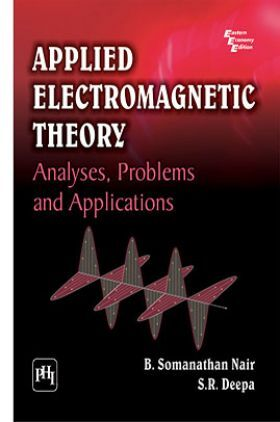# Applied Electromagnetic Theory : Analyses,Problems And Applications

Sold ( 51 times )
7560 Views

This product is currently not available.

Save extra with 1 Offers

Get ₹ 50

Instant Cashback on the purchase of ₹ 400 or above

Product Specifications

 Publisher PHI Learning All IES Electrical Engineering books by PHI Learning ISBN 9788120333390 Author: NAIR, B. SOMANATHAN, DEEPA, S. R. Number of Pages 384 Available Available in all digital devicesAbout The Book Applied Electromagnetic Theory

Book Summary:

Designed as a textbook for the students of electronics and communi-cation engineering, and electrical and electronics engineering, it covers the subject of electromagnetism with a clear exposition of the theory in association with the practical applications. The text explains the physical and mathematical aspects of the highly complicated electromagnetic theory in a very simple manner.

The book begins with a introductory chapter on vector theory and then moves on to explain the effectiveness of Amperes circuital law and Biot-Savarts law in dealing with magnetostatic problems, derivation of Maxwells field equations from the fundamental laws of Faraday and Ampere, free-space solutions of wave equations, and the theory of skin effect. Finally, it concludes with the applications of Smith chart in solving transmission line problems and the theory of rectangular and circular waveguides.

Key Features

Large number of solved examples and chapter-end problems

Appendices to give the solutions of wave equations in waveguides

Three-dimensional figures to illustrate theories

Generalized solution of Maxwells equations

Besides undergraduate students of engineering, it would be useful for the postgraduate students of physics.

Preface
0 Introduction to Vector Theory
MODULE I
1 Coulombs Law and Its Applications
2 Poissons and Laplaces Equations
3 Magnetostatics
4 Maxwells Equations
5 Poyntings Theorem
MODULE II
6 Electromagnetic Waves
7 Reflection and Refraction of Plane Waves
8 Uniform Transmission Lines
MODULE III
9 Impedance Matching Using Stub Lines
10 Rectangular and Circular Waveguides
Appendices
A:Transverse Electric (TE) Waves in Rectangular Waveguides
B:Circular Cylindrical Waveguides
C:Properties of Materials
Bibliography Index